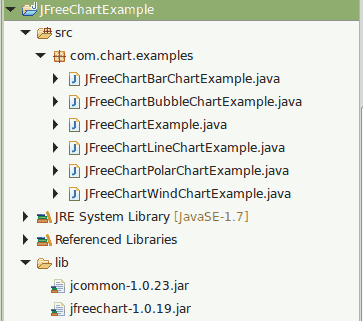# Create Scatter Chart Using JFreeChart

Create Scatter Chart Using JFreeChart explains about creating a simple scatter chart using JFreechart API

A scatter plot (also called a scatterplot, scatter graph, scatter chart, scattergram, or scatter diagram) is a type of plot or mathematical diagram using Cartesian coordinates to display values for typically two variables for a set of data. If the points are color-coded, one additional variable can be displayed. The data are displayed as a collection of points, each having the value of one variable determining the position on the horizontal axis and the value of the other variable determining the position on the vertical axis.

Reference -> https://en.wikipedia.org/wiki/Scatter_plot

How To Create Scatter Chart Using JFreeChart library?

JFreeChart is a free and open source java chart library used for creating professional quality charts. JFreeChart is purely written in java language, we can very easily incorporate JFreeChart in our java standalone and web applications.

Referenece - > http://www.jfree.org/jfreechart/

Here I am showing an example about How to create  a simple Scatter Chart using JFreeChart.

Note

1) If you need pie chart example, You can follow Create Pie Chart Using JFreeChart

2) If you need line chart example, You can follow Create Line Chart Using JFreeChart

3) If you need bar chart example, You can follow Create Bar Chart Using JFreeChart

4) If you need polar chart example, You can follow Create Polar Chart Using JFreeChart

5) If you need bubble chart example, You can follow Create Bubble Chart Using JFreeChart

6) If you need wind chart example, You can follow Create Wind Chart Using JFreeChart

###### Required Libraries

Following jar must be in classpath

1. jfreechart-1.0.19.jar
2. jcommon-1.0.23.jar

We can create following professional quality charts by using jfreechart.

• Single valued Charts such as compass, speedometer, thermometer
• Line Chart
• Pie Chart
• Bar Chart
• Bubble Chart
• Wind Chart
• Polar Chart

## Project Structure